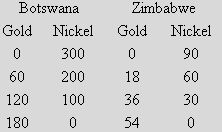### CFA Practice Question

There are 923 practice questions for this topic.

### CFA Practice Question

Refer to the table below. A comparative advantage in the production of nickel is held by ______.A. Zimbabwe
B. Botswana
C. neither country

Since both countries have the same opportunity cost of producing nickel, neither country has a comparative advantage in nickel production.

User Comment
lna1717 but Botswana produces 300 vs 0 and Zimbabwe 90 vs 0?
cbb1 For comparative advantage, need to look at ratios when both are producing.
theBooty think about slope of the line
Done Divide the production of nickel over gold. You will see the trend. This is about units of production....I think?
(0+60+120+180) = Botswana Gold = 360
(300+200+100+0) = Botswana Nickel = 600
opportunity cost = 360/600 =0.6
(0+18+36+54) = Zimbabwe Gold = 108
(90+60+30+0) = Zimbabwe Nickel = 180
opportunity cost = 108/180 =0.6
Same opportunity cost, No Comparative advantage.
Shelton y_1=(-5/3)x_1 + 300, y_2=(-5/3)x_2+90 => same opp cost = 5/3
same opp cost of producing 5/3 units of Nickel per unit of Gold
Bibhu There is another short cut to answer the question. Just get slope of the line i.e. (y2-y1)/(x2-x1) e.g.200-300/60-0=-100/60=-5/3 for Botswana. For Zimbabwe its 60-90/18-0=-5/3. So there is no comparative advantage. If they had asked for comparative advantage of gold y should be replaced by gold values.
ljamieson faster: 300/180 = 5/3 = 90/54
Smiley225 good question!
Yrazzaq88 I did it this way:

90+60+30 = 180
90/180= .5

300+200+100=600

300/600 = .5

both are the same, as per the highest output that they can produce.
chesschh opportunity cost has to be involved. 0 gold would not apply
choas69 wtf ?
200/60=0.33
60/18=0.33
kseeba17 Not sure why some people seem to make everything so darn complicated, just use your common sense guys.

Zim can produce 90 nickel or 54 silver ... 90/54 = 1.66Nickel per silver

Bowstwana can produce 300 nickel or 180 silver ... 300/180 = 1.66 Nickel per silver

AKA the same.
maryprz14 300/180 = 90/54